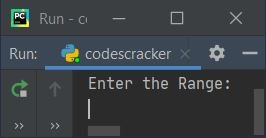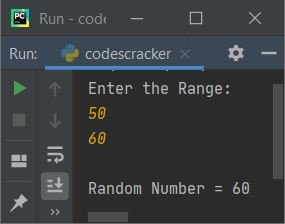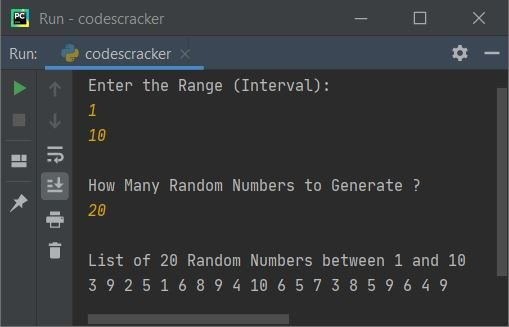# Python Program to Generate Random Numbers

This article is created to cover some programs in Python, that generate and prints random numbers. Here are the list of programs on generating random numbers in Python:

• Generate a single random number
• Generate a random number in given range
• Generate multiple random numbers in given range

## Generate a Random Number

This program generate and prints a single random number using random.random(). The function random() is defined in random module, therefore we've to import the module before calling the function, like shown in the program given below:

```import random

randnum = random.random()
print("Random Number =", randnum)```

Here is its sample output:## Generate a Random Number in Given Range

This program also does the same job of generating random number, but in a given range. The range must be provided by user at run-time. For example, if user enters range as 10 and 40, then random number generated will be greater than or equal to 10 and less than or equal to 40.

```import random

print("Enter the Range: ")
start = int(input())
end = int(input())

randnum = random.randint(start, end)
print("\nRandom Number =", randnum)```

Here is its sample run:Now supply the input say 50 and 60 as range to generate a random number lies between these two numbers like shown in the snapshot given below:#### Modified Version of Previous Program

This program uses try-except to handle with invalid inputs. Rest of the things are similar to previous program.

```import random

print("Enter the Range: ")
try:
start = int(input())
try:
end = int(input())
randnum = random.randint(start, end)
print("\nRandom Number =", randnum)
except ValueError:
print("\nInvalid Input!")
except ValueError:
print("\nInvalid Input!")```

This program produces same output as of previous program.

## Generate Multiple Random Numbers in Given Range

To generate multiple random numbers in given range in Python, you have to ask from user to enter the range, then ask to enter the value of n to print n random numbers in given range, like shown in the program given below:

The question is, write a Python program to generate and print n random numbers in given range. Here is its answer:

```import random

print("Enter the Range (Interval): ")
a = int(input())
b = int(input())

if a>b:
t = a
a = b
b = t

print("\nHow Many Random Numbers to Generate ? ")
tot = int(input())

randnums = []
for i in range(tot):
randnums.append(random.randint(a, b))

print("\nList of " +str(tot)+ " Random Numbers between " +str(a)+ " and " +str(b))
for i in range(tot):
print(randnums[i], end=" ")
print()```

Here is its sample run with user input 1 and 10 as range, and 20 as size to generate twenty random numbers between 1 and 10 like shown in the snapshot given below:The statement (from above program) randnums = [] defines a empty list. The following code:

`for i in range(tot):`

is applied to execute the following statement:

`randnums.append(random.randint(a, b))`

tot number of times. The append() method appends or adds an element at the end of list randnums. for example, if tot=10, then 10 random numbers gets appended to the list named randnums one by one

The str() method is used to convert from int to string type, so that, using +, all the strings inside print() gets concatenated and printed at once, on output screen.

#### Same Program in Other Languages

Python Online Test

« Previous Program Next Program »